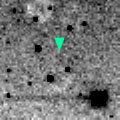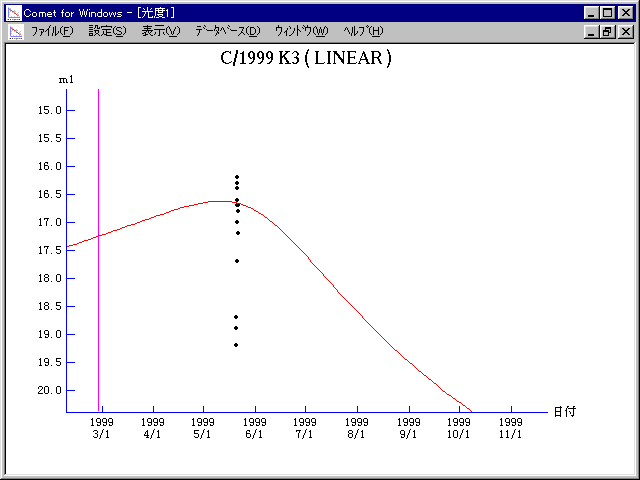# \$B%j%K%"WB@1(B

C/1999 K3 ( LINEAR )###\$B%W%m%U%#!<%k(B

 \$BH/8+F|(B 1999\$BG/(B 5\$B7n(B20\$BF|(B \$BH/8+8wEY(B 18.7\$BEy(B \$BH/8+ Lincoln Laboratory Near-Earth Asteroid Research project

###\$B###\$B50F;MWAG(B

```   The following improved orbital elements by Kenji Muraoka, are
from 52 observations  1999 May 20  to  July 18, perturbations
by 9 Planets, Moon and 5 minor planets were taken into account.
The mean residual is +/- 0.74 arc seconds.

Epoch  =  1999 Mar.  3.0  TT       JDT = 2451240.5
T  =  1999 Feb. 27.53047       +/- 0.00862 (m.e.) TT
Peri. =  341.14802                +/- 0.00441
Node  =  266.91301                +/- 0.00093   (2000.0)
Incl. =   92.27261                +/- 0.00205
q  =    1.9287637              +/- 0.0000679 AU
e  =    0.9917448              +/- 0.0001938
1/a  =   +0.0042800              +/- 0.0001005 1/AU
( P  =   3571 years )
orig. 1/a  =   +0.004165
fut.  1/a  =   +0.004955
```

###\$B@1?^(B###\$B8wEYJQ2=(B

```        m1 = 12.5 + 5 log\$B&\$(B + 10.0 log r
```##### \$B50F;MWAG\$OB<2,7r<#;a\$N7W;;\$K\$h\$k\$b\$N\$G\$9!#(B \$B@1?^\$O(B StellaNavigator Ver.2.0 for Windows (\$B%"%9%H%m%"!<%D(B \$BJTCx(B / \$B%"%9%-!<=PHG6I4)(B) \$B\$G:n@.\$7\$?\$b\$N\$G\$9!#(B \$B8wEY%0%i%U\$O(BComet for Windows\$B\$G:n@.\$7\$?\$b\$N\$G\$9!#(B